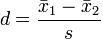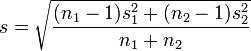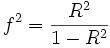# Effect Size

Effect size is a statistical concept that measures the strength of the relationship between two variables on a numeric scale.  For instance, if we have data on the height of men and women and we notice that, on average, men are taller than women, the difference between the height of men and the height of women is known as the effect size.  The greater the effect size, the greater the height difference between men and women will be.  Statistic effect size helps us in determining if the difference is real or if it is due to a change of factors.  In hypothesis testing, effect size, power, sample size, and critical significance level are related to each other.  In Meta-analysis, effect size is concerned with different studies and then combines all the studies into single analysis.  In statistics analysis, the effect size is usually measured in three ways: (1) standardized mean difference, (2) odd ratio, (3) correlation coefficient.Types of effect size

Pearson r correlation: Pearson r correlation was developed by Karl Pearson, and it is most widely used in statistics.  This parameter of effect size is denoted by r.  The value of the effect size of Pearson r correlation varies between -1 to +1.  According to Cohen (1988, 1992), the effect size is low if the value of r varies around 0.1,  medium if r varies around 0.3, and large if r varies more than 0.5.  The Pearson correlation is computed using the following formula:Where
r = correlation coefficient
N = number of pairs of scores
∑xy = sum of the products of paired scores
∑x = sum of x scores
∑y = sum of y scores
∑x2= sum of squared x scores
∑y2= sum of squared y scores

Standardized means difference: When a research study is based on the population mean and standard deviation, then the following method is used to know the effect size:The effect size of the population can be known by dividing the two population mean differences by their standard deviation.

Cohen’s d effect size: Cohen’s d is known as the difference of two population means and it is divided by the standard deviation from the data. Mathematically Cohen’s effect size is denoted by:Where s can be calculated using this formula:Glass’s Δ method of effect size: This method is similar to the Cohen’s method, but in this method standard deviation is used for the second group.  Mathematically this formula can be written as:Hedges’ g method of effect size: This method is the modified method of Cohen’s d method. Hedges’ g method of effect size can be written mathematically as follows:Where standard deviation can be calculated using this formula:Cohen’s f2 method of effect size: Cohen’s f2 method measures the effect size when we use methods like ANOVA, multiple regression, etc.  The Cohen’s f2 measure effect size for multiple regressions is defined as the following:Where R2 is the squared multiple correlation.

Cramer’s φ or Cramer’s V method of effect size: Chi-square is the best statistic to measure the effect size for nominal data.  In nominal data, when a variable has two categories, then Cramer’s phi is the best statistic use.  When these categories are more than two, then Cramer’s V statistics will give the best result for nominal data.

Odd ratio: The odds ratio is the odds of success in the treatment group relative to the odds of success in the control group.  This method is used in cases when data is binary.  For example, it is used if we have the following table:

 Frequency Success Failure Treatment group a b Control group c d

To measure the effect size of the table, we can use the following odd ratio formula:Related Pages: